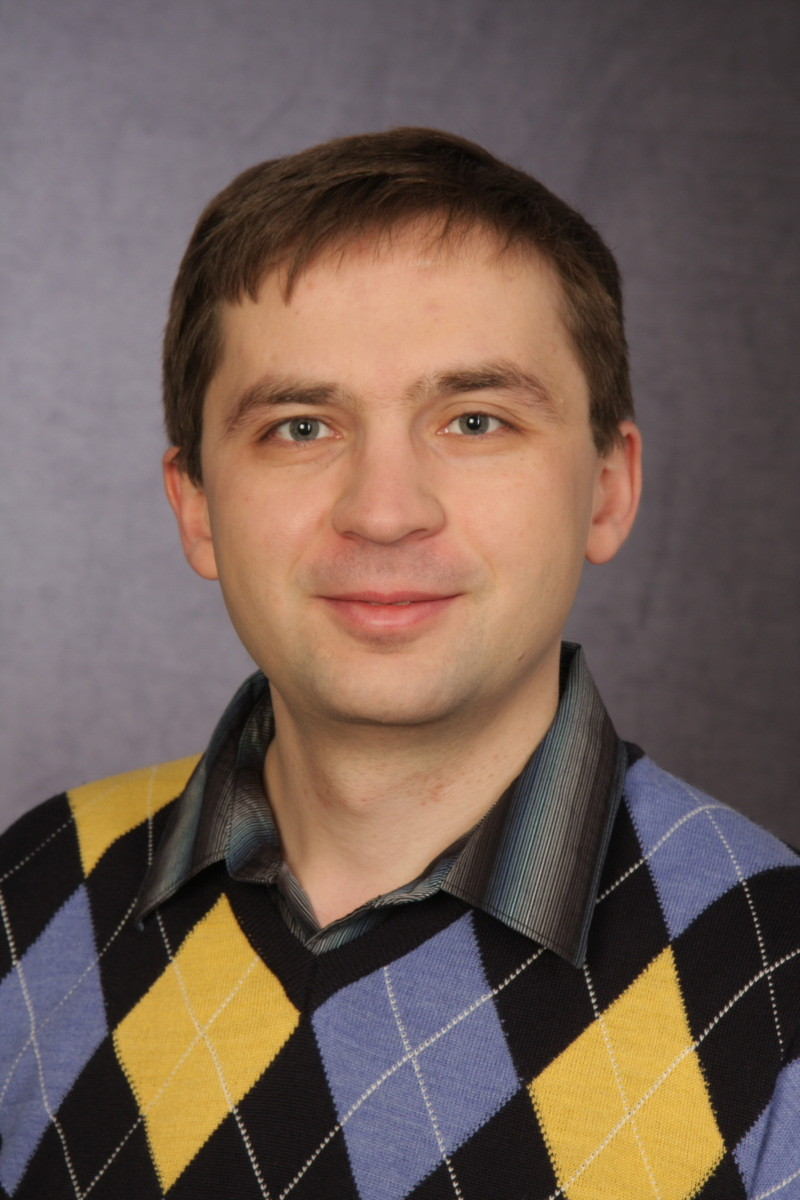# Prof. Dr. Igor Burban## Research Interests

• Homological algebra, derived and triangulated categories
• Vector bundles and coherent sheaves on singular curves
• Ringel-Hall algebras and quantum affine algebras
• Representation theory of associative algebras
• Fourier-Mukai transforms
• Cohen-Macaulay modules
• Yang-Baxter equations
NEWS : On 1. April 2018, I have moved to the University of Paderborn. Here ist a link to my new web page .

## Recent preprints

• I.Burban, Yu.Drozd, On the derived categories of gentle and skew-gentle algebras: homological algebra and matrix problems (pdf), arXiv:1706.08358.
• I.Burban, A.Zheglov, Cohen-Macaulay modules over the algebra of planar quasi-invariants and Calogero-Moser systems (pdf), arXiv:1703.01762.
• I.Burban, L.Galinat, Torsion free sheaves on Weierstraß cubic curves and the classical Yang-Baxter equation (pdf), arXiv:1612.04586.
• I.Burban, A.Zheglov, Fourier-Mukai transform on Weierstraß cubics and commuting differential operators (pdf), arXiv:1602.08694.

## Recent publications

• I.Burban, L.Galinat, A.Stolin, Simple vector bundles on a nodal Weierstraß cubic and quasi-trigonometric solutions of CYBE (pdf), to appear in Journal of Physics A: Mathematical and Theoretical doi: 10.1088/1751-8121/aa8e35, see also arXiv:1704.07202.
• I.Burban, Yu.Drozd, Maximal Cohen-Macaulay modules over non-isolated surface singularities and matrix problems (pdf), Memoirs of the AMS, vol. 248, no. 1178 (2017).
• I.Burban, Yu.Drozd, V.Gavran, Minors of non-commutative schemes (pdf), European Journal of Math. (2017), doi: 10.1007/s40879-017-0128-6.
• I.Burban, Yu.Drozd, V.Gavran, Singular curves and quasi-hereditary algebras (pdf), Int. Math. Res. Notices, vol. 2017, no. 3, 895-920 (2017).
• I.Burban, W.Gnedin, Cohen-Macaulay modules over some non-reduced curve singularities (pdf), Journal of Pure and Applied Algebra, vol. 220, no. 12, 3777-3815 (2016).
• I.Burban, T.Henrich, Vector bundles on plane cubic curves and the classical Yang-Baxter equation (pdf), Journal of the European Math. Society, vol. 17, no. 3, 591-644 (2015).
• I.Burban, B.Kreussler, Analytic moduli spaces of simple sheaves on families of integral curves (pdf), Math. Nachr., vol. 287, no. 2-3, 173-183 (2014).
• I.Burban, O.Schiffmann, Composition algebra of a weighted projective line (pdf), Journal für Reine und Angew. Mathematik, vol. 679, 75-124 (2013).
• I.Burban, M.Kalck, Relative singularity category of a non-commutative resolution of singularities (pdf), Adv. in Math., vol. 231, no. 1, 414-435 (2012).
• I.Burban, Frobenius morphism and vector bundles on cycles of projective lines (pdf), Comm. in Algebra, vol. 40, no. 8, 2983-2988 (2012).
• I.Burban, T.Henrich, Semi-stable vector bundles on elliptic curves and the associative Yang-Baxter equation (pdf), Journal of Geometry and Physics, vol. 62, no. 2, 312-329 (2012).
• I.Burban, B.Kreussler, Vector bundles on degenerations of elliptic curves and Yang-Baxter equations (pdf), Memoirs of the AMS, vol. 220, no. 1035 (2012).
• I.Burban, O.Schiffmann, Two descriptions of the quantum affine algebra \$U_v(sl^_2)\$ via Hall algebra approach (pdf), Glasgow Mathematical Journal, vol. 54, no. 2, 283-307 (2012).
• I.Burban, O.Schiffmann, On the Hall algebra of an elliptic curve I (pdf), Duke Math. Journal, vol. 161, no. 7, 1171-1231 (2012).
• I.Burban, Yu.Drozd, Tilting on non-commutative rational projective curves (pdf), Mathematische Annalen, vol. 351, no. 3, 665-709 (2011).

## Lecture Notes

• Coherent sheaves on an elliptic curve (pdf), Notes of Kristian Bruening of my lecture course given at Tsinghua University in August 2005, Beijing, China.
• Du Val Singularities (pdf)
• Maximal Cohen-Macaulay modules over quotient surface singularities (pdf). These are notes of my talks given in Paderborn and Osaka in 2009.